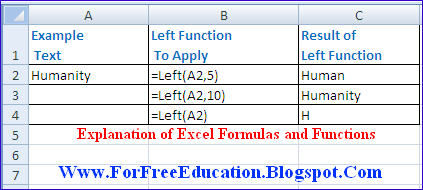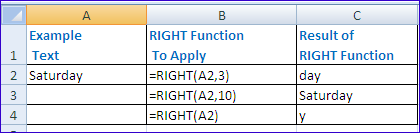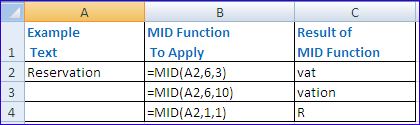# Uses of Left Right Mid Text Functions in Excel

•
•
•
•
•
•
•
•
•
•

## Why To Use Left Function in Microsoft Excel?

The Left function is used to get first N characters from the given text string or the text contained in a cell.

### Syntax of LEFT Function in Excel

LEFT(Text, Number_of_characters)

For example, the function LEFT(“Normal”,3) will return Nor.

### Syntax of LEFT function with Cell Reference

LEFT(CellReference, Number_of_characters)

For example, let the cell A2 contains the text Humanity, the function LEFT(A2,5) will return Human.
Similarly if Cll A12 contains “England” then Left(A12,5) will give “Engla” that is first five characters of contents of cell A12 from left side.

### How To Implement LEFT Function in ExcelUses of Excel Functions Left Right in Excel Worksheet

First of all place the cursor in an empty cell next to cell A2, to enter the function.

• Type =
• Type the function name LEFT
• Type the left parenthesis (
• Type A2 from keyboard.
• Type , (that is Comma)
• Type 5 so that the first 5 characters will be returned from cell A2’s text.
• Press Enter key (You need not to enter right parenthesis, it will be automatically included)
• The result Human will be displayed in the cell.

Note: Number_of_characters will be normally greater than zero. If Number_of_characters will greater than the total length of the text then All the text will be returned. For example, in above example the function LEFT(A2, 10) will return the whole text in cell A2 that is Humanity.
Similarly, if we ignore the value of Number_of_characters then it is assumed to be 1 by default. So that the function LEFT(A2) will return only one character that is H.

### Why To Use Right Function in Microsoft Excel?

The Right function is used to get the last N characters in a text string. Where N is the number of characters to be extracted from the given string.

### Syntax of RIGHT Function in Excel

RIGHT(Text, Number_of_characters)

For example, the function Right(“Night”,2) will return ht.

### Syntax of RIGHT function with Cell Reference

RIGHT(CellReference, Num_of_char)

For example, let the cell A2 contains the text Saturday, the function RIGHT(A2,3) will return day.

### How To Implement RIGHT Function in ExcelFirst of all place the cursor in an empty cell next to cell A2, to enter the function.

• Type =
• Type the function name RIGHT
• Type the left parenthesis (
• Type A2 from keyboard.
• Type , (that is Comma)
• Type 3 so that the last 3 characters will be returned from cell A2’s text.
• Press Enter key (You need not to enter right parenthesis, it will be automatically included)
• The result day will be displayed in the cell.

Note:

• Num_of_char will be greater than zero normally.
• If Num_of_char is greater than the length of given text, then RIGHT function will return all of text.
• If the value of Num_of_char is not used then its default value 1 is used and only one last character is returned by the RIGHT function.

## Why To Use MID Function in Microsoft Excel?

The MID function is used to get the last N characters in a text string. Where N is the number of characters to be extracted from the given string.

### Syntax of RIGHT Function in Excel

MID(text, start_num, num_chars)

For example, the function MID(“Night”,1,3) will return Nig.

### Syntax of RIGHT function with Cell Reference

RIGHT(CellReference, start_num, num_chars)

Where start_num is the number of starting character and num_chars is total number of characters to be extracted from the text.
For example, let the cell A2 contains the text Reservation,

• the function MID(A2,6,3) will return vat.
• the function MID(A2,6,10) will return vation. Since 10 is greater than the length of 6 th character to end of text, so this MID function will return all text starting from 6th character v to last character n.
• the function MID(A2,1,1) will return only one character starting from position 1 that is R.

### How To Implement RIGHT Function in ExcelFirst of all place the cursor in an empty cell next to cell A2, to enter the function.

• Type =
• Type the function name MID
• Type the left parenthesis (
• Type A2 from keyboard.
• Type , (that is Comma)
• Type 6
• Type ,
• Type 3 so that the 3 characters from position 6 will be returned cell A2’s text.
• Press Enter key (You need not to enter right parenthesis, it will be automatically included)
• The result vat will be displayed in the cell.